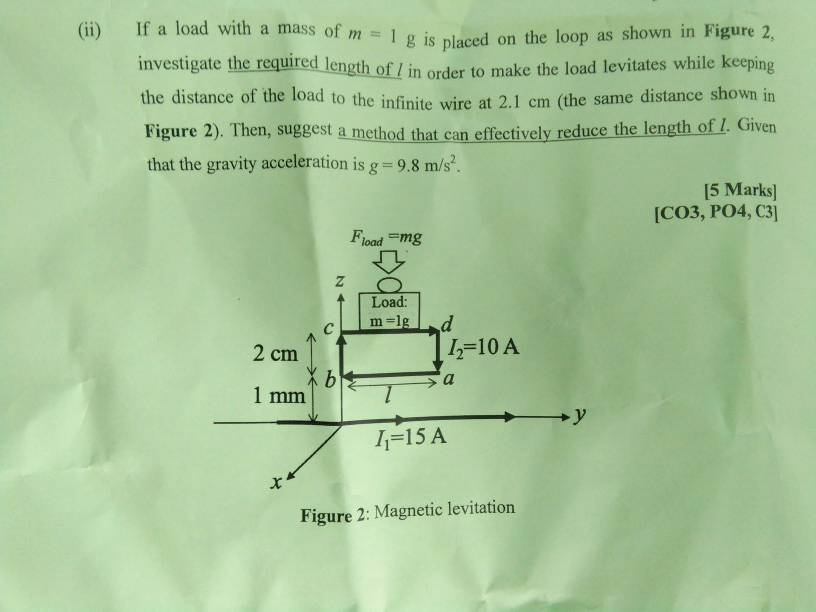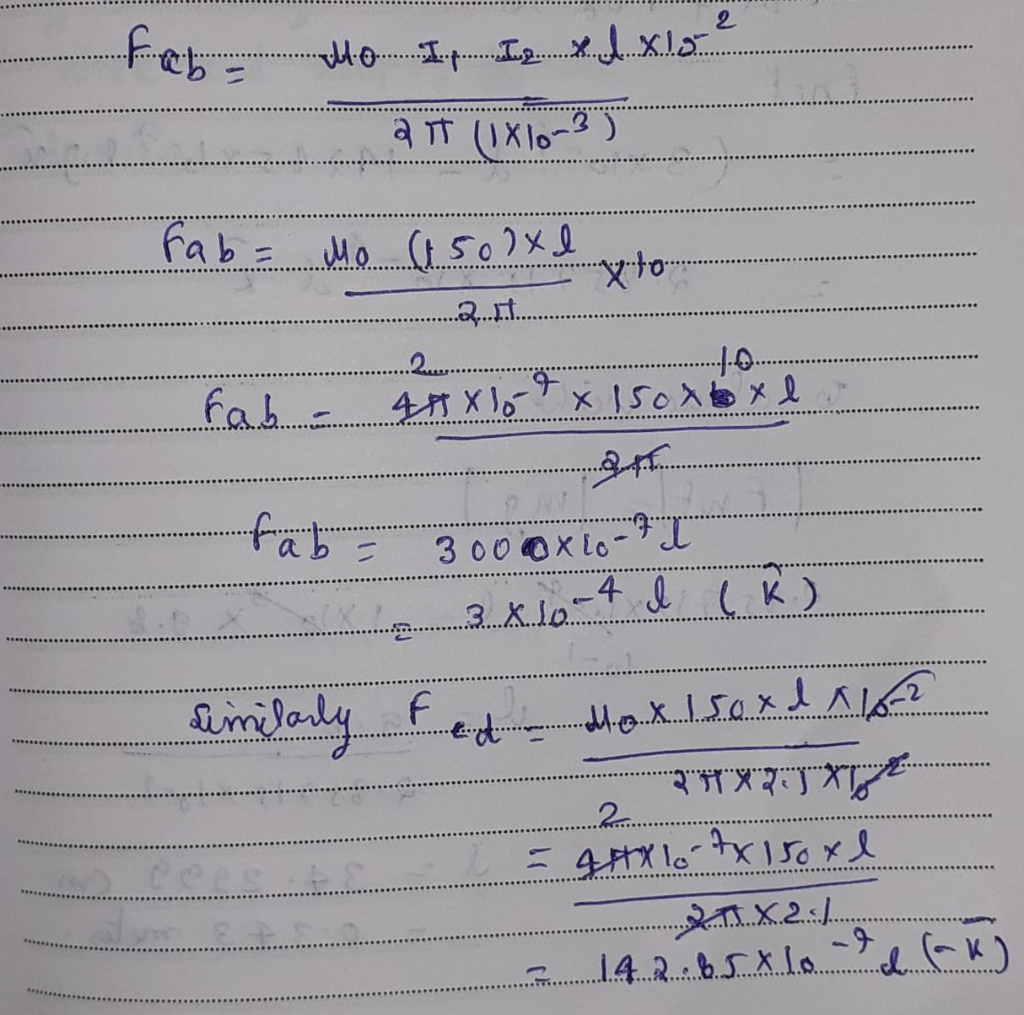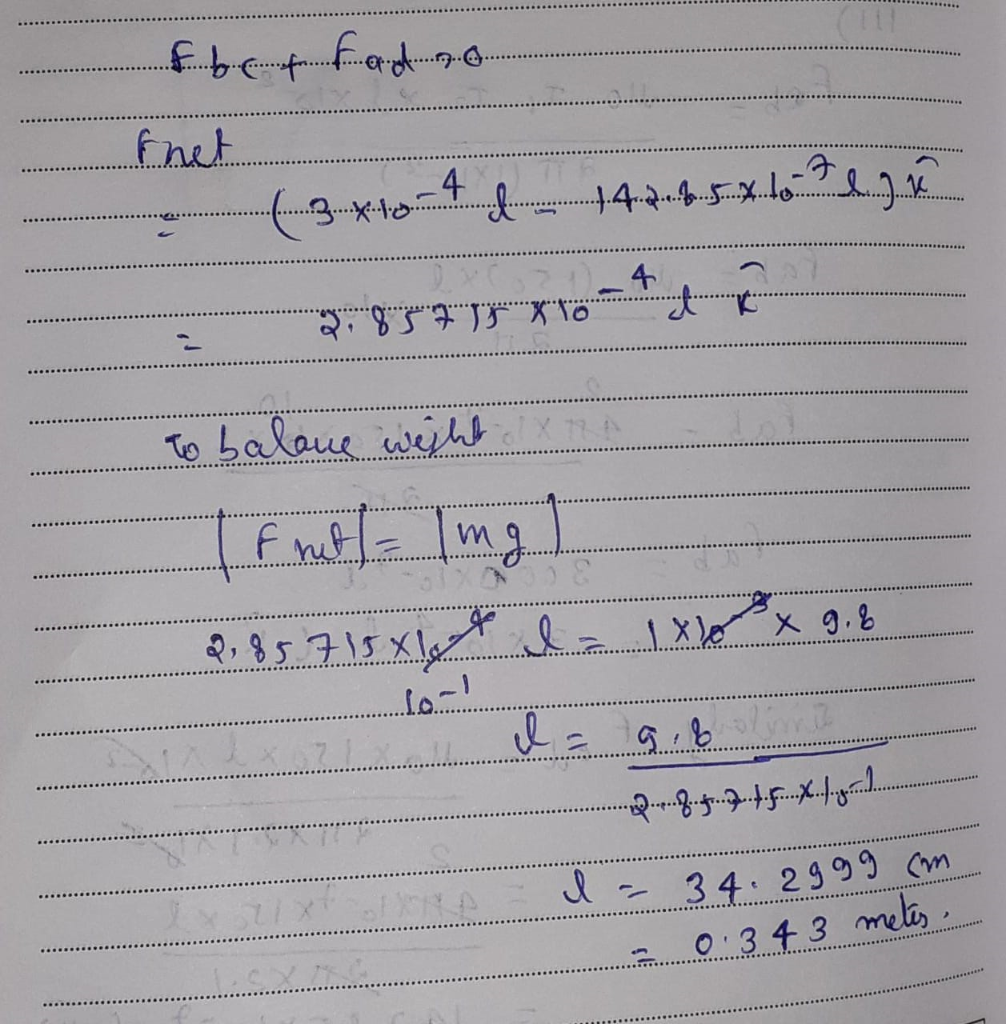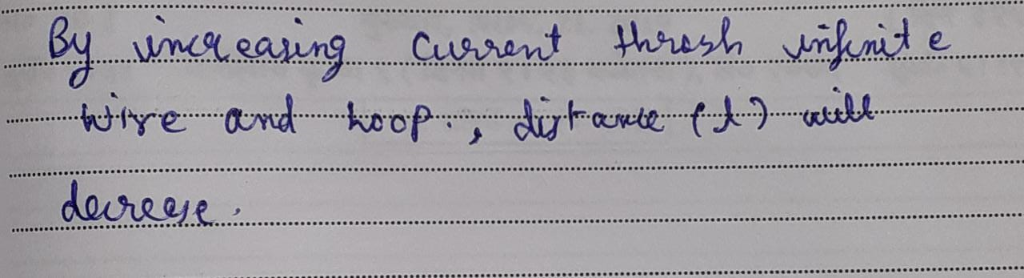# electromagnetic fields theory If a load with a mass of m Ig is placed on the loop as shown in Figure 2, investigate...electromagnetic fields theory

If a load with a mass of m Ig is placed on the loop as shown in Figure 2, investigate the required length of 1 in order to make the load levitates while keeping (ii) the distance of the load to the infinite wire at 2.1 cm distance shown in (the same Figure 2). Then, suggest a method that can effectively reduce the length of l. Given that the gravity acceleration is g 9.8 m/s2. 15 Marks] [CO3, PO4, C3] Fload mg Z Load: m-1g d =10 A C 2 cm X b 1 mm a +y -15 A Figure 2: Magnetic levitation##### Add Answer of: electromagnetic fields theory If a load with a mass of m Ig is placed on the loop as shown in Figure 2, investigate...
More Homework Help Questions Additional questions in this topic.